Here is this month's selection of Traders' Tips, contributed by various developers of technical analysis software to help readers more easily implement some of the strategies presented in this and other issues.

You can copy these formulas and programs for easy use in your spreadsheet or analysis software. Simply "select" the desired text by highlighting as you would in any word processing program, then use your standard key command for copy or choose "copy" from the browser menu. The copied text can then be "pasted" into any open spreadsheet or other software by selecting an insertion point and executing a paste command. By toggling back and forth between an application window and the open Web page, data can be transferred with ease.

This month's tips include formulas and programs for: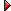TRADESTATION: Fractal Adaptive Moving AverageMETASTOCK: Fractal Adaptive Moving AverageAIQ EXPERT DESIGN STUDIO: Fractal Adaptive Moving AverageWEALTH-LAB: Fractal Adaptive Moving AverageeSIGNAL: Fractal Adaptive Moving AverageNEUROSHELL TRADER: Fractal Adaptive Moving AverageAMIBROKER: Fractal Adaptive Moving AverageNEOTICKER: Fractal Adaptive Moving AverageTRADINGSOLUTIONS: Fractal Adaptive Moving AverageFINANCIAL DATA CALCULATOR: Fractal Adaptive Moving Average

John Ehlers' article in this issue, "Fractal Adaptive Moving Averages," already presents some EasyLanguage code for an adaptive moving average. This adaptive moving average is based on the fractal properties of a price series.

We have converted Ehlers' code for this moving average into an EasyLanguage function, so that it can be called from any indicator or strategy. The function's name is "AdaptMovAvg_Fractal."

We have also adapted an existing strategy based on Bollinger Bands so that it calls this new function. The revised Bollinger Band strategy is called "FractalAMA Bands." It calls "AdaptMovAvg_Fractal" for both the variance and band calculations.

```Strategy:  FractalAMA Bands
{ The IntrabarOrderGeneration attribute is set to false in this strategy because
strategy calculations depend on end-of-bar prices. }
[IntrabarOrderGeneration = false]
inputs:
Price( 0.5 * ( H + L ) ),
N( 16 ),
NumDevsUp( 2 ),
NumDevsDn( 2 ),
TrailAmt( 0.5 ),
BandColor( Yellow ),
DataType( 1 ) ; { pass in 1 if you are working with the entire population, and 2
if you are working with a sample and want the standard deviation
for the population }
variables:
Divisor( 0 ),
Mean( 0 ),
SumSqr( 0 ),
AvgDiff( 0 ),
FracStandardDev( 0 ),
LowerBand( 0 ),
UpperBand( 0 ),
TL_ID1( -1 ),
TL_ID2( -1 ) ;
// AvgDiff = 0 ;
Divisor = Iff( DataType = 1, N, N - 1 ) ;
if Divisor > 0 then
begin
Mean = AdaptMovAvg_Fractal( Price, N ) ;
SumSqr = 0 ;
for Value1 = 0 to N - 1
begin
SumSqr = SumSqr + Square( Price[Value1] - Mean ) ;
end ;
AvgDiff = SumSqr / Divisor ;
end ;
if AvgDiff > 0 then
FracStandardDev = SquareRoot( AvgDiff )
else
FracStandardDev = 0 ;
LowerBand = Mean - NumDevsDn * FracStandardDev ;
UpperBand = Mean + NumDevsUp * FracStandardDev ;
if CurrentBar > 1 and Low crosses over LowerBand then
{ CB > 1 check used to avoid spurious cross confirmation at CB = 1 }
Buy ( "BBandLE" ) next bar LowerBand stop ;
if CurrentBar > 1 and High crosses under UpperBand then
SellShort next bar at UpperBand Stop ; { ie, don't buy if next bar completely
below current LowerBand, but wait for
next crossing condition - an example of
a non-persistent setup with an
indefinite stop/limit trigger }
SetStopShare ;
SetDollarTrailing( TrailAmt ) ;
SetProfitTarget ( 3 * TrailAmt ) ;
{ Use trendlines to "plot" the bands on the chart }
TL_ID1 = TL_New( Date, Time, UpperBand, Date,
Time, UpperBand ) ;
TL_SetColor( TL_ID1, BandColor ) ;
TL_ID2 = TL_New( Date, Time, LowerBand, Date,
Time, LowerBand ) ;
TL_SetColor( TL_ID2, BandColor ) ;
inputs:
Price( NumericSeries ),
N( NumericSimple ) ; { N must be an even number }
variables:
N3( 0 ),
HH( 0 ),
LL( 0 ),
HalfN( 0 ),
Count( 0 ),
N1( 0 ),
N2( 0 ),
Dimen( 0 ),
Alpha( 0 ),
Filt( 0 ) ;
N3 = ( Highest( High, N ) - Lowest( Low, N ) ) / N ;
HH = High ;
LL = Low ;
HalfN = 0.5 * N ;
for Count = 0 to HalfN - 1
begin
if High[Count] > HH
then HH = High[Count] ;
if Low[Count] < LL then
LL = Low[Count] ;
end ;
N1 = ( HH - LL ) / HalfN ;
HH = High[HalfN] ;
LL = Low[HalfN] ;
for Count = HalfN to N - 1
begin
if High[Count] > HH then
HH = High[Count] ;
if Low[Count] < LL then
LL = Low[Count] ;
end ;
N2 = ( HH - LL ) / HalfN ;
if N1 > 0 and N2 > 0 and N3 > 0 then
Dimen = ( Log( N1 + N2 ) - Log( N3 ) ) / Log( 2 ) ;
Alpha = ExpValue( -4.6 * ( Dimen - 1 ) ) ;
if Alpha < 0.01 then
Alpha = 0.01
else if Alpha > 1 then
Alpha = 1 ;
Filt = Alpha * Price + ( 1 - Alpha ) * Filt ;
if CurrentBar < N + 1 then
Filt = Price ;
This code and function will be available for download from the Support Center at TradeStation.com. Search for the file "Frama.eld." Ehlers' original code can be found in the .eld file.
--Mark Mills
EasyLanguage Questions Forums
A subsidiary of TradeStation Group, Inc.
GO BACK

John Ehlers' article in this issue, "Fractal Adaptive Moving Averages," introduces an indicator of the same name. In his indicator formula, he restricts the number of periods to an even number. The formula in MetaStock avoids this restriction by asking for the smaller time frame. This number is then used for the two half-interval calculations and is then doubled for the full interval calculation. The formula for this indicator and the steps to include it in MetaStock are presented here.

To enter this indicator into MetaStock:

```1. In the Tools menu, select Indicator Builder.
2. Click New to open the Indicator Editor for a new indicator.
3. Type the name of the formula.
4. Click in the larger window and input the following formula:```
```Name:  FRAMA
Formula:
y:=Input("sample time periods",1,20,8);
y2:=2*y;
n1:=(HHV(H,y)-LLV(L,y))/y;
n2:=Ref((HHV(H,y)-LLV(L,y))/y,-y);
n3:=(HHV(H,y2)-LLV(L,y2))/y2;
x:=(Log(n1+n2)-Log(n3))/Log(2);
xt:=Exp(-4.6*(x-1));
x1:=If(xt<0.1,0.1,If(xt>1,1,xt));
x2:=1-x1;
If(Cum(1)=y2,
(MP() * x1) + (Ref(MP(),-1) * x2),
(MP() * x1) + (PREV * x2))```
--William Golson, Equis International
www.equis.com
GO BACK

AIQ EXPERT DESIGN STUDIO: Fractal Adaptive Moving Average

The AIQ code for John Ehlers' fractal adaptive moving average (FRAMA) is shown here together with two sample trading systems that we used in a backtest to determine if the FRAMA is an improvement over a fixed-period exponential moving average.

A value of N=40 was used to run the FRAMA test. The exponential average test was run using a fixed period of 40 days. The systems buy when the price crosses above the moving average and sell when the price crosses below the moving average. Only the long side was tested.

Figure 1 shows a comparison of a FRAMA with N=40 to an exponential moving average for 40 days. The FRAMA is more responsive to price changes than the exponential moving average. The backtest results shown in Figure 2, which were run on the NASDAQ 100 list of stocks, show that the FRAMA is an improvement over the exponential moving average for the sample trading system tested.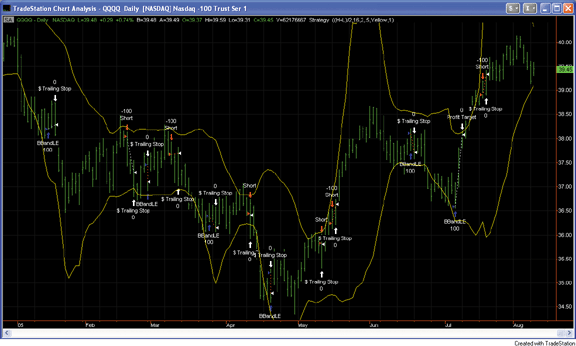FIGURE 1: TRADESTATION, QQQQ. Here's a sample TradeStation daily bar chart demonstrating the fractal adaptive moving average. For clarity's sake, the FRAMA indicator line is not shown.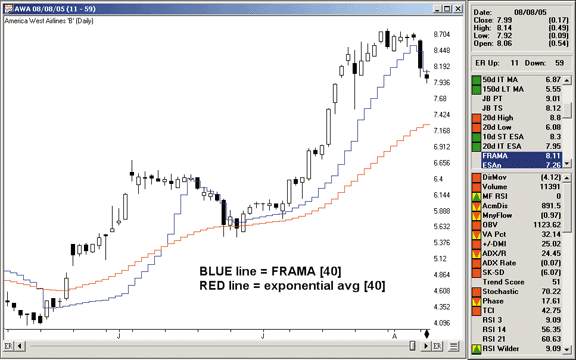FIGURE 2: AIQ EXPERT DESIGN STUDIO, FRAMA. Here is a comparison of FRAMA with N=40 to an exponential moving average for 40 days. The FRAMA appears to be more responsive to price changes than the exponential moving average.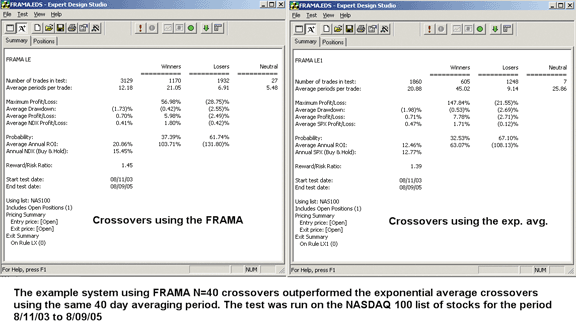FIGURE 3: AIQ EXPERT DESIGN STUDIO, BACKTEST RESULTS FOR FRAMA. The backtest results based on the NASDAQ 100 list of stocks show that the FRAMA is an improvement over the exponential moving average for this sample trading system.

The AIQ code is shown here but can also be downloaded from www.aiqsystems.com/S&C1.htm.

```!! FRAMA Fractal Adaptive Moving Average
!! Author: John Ehlers
!! Coded by Richard Denning 8/9/05
!CODING ABREVIATIONS:
H is [high].
L is [low].
C is [close].
O is [open].
Price is  (H+L)/2.
Define N   40.    !MUST BE AN EVEN NUMBER
N3  is (highresult(H,N,0) - lowresult(L,N,0)) / N.
N1  is (highresult(H,N / 2 - 1,0)
- lowresult(L,N / 2 - 1,0)) / (N / 2).
N2  is (highresult(H,N / 2,N / 2 - 1)
- lowresult(L,N / 2,N / 2 - 1)) / (N / 2).
Dimen is iff(N1 > 0 and N2 > 0
and N3 > 0,(ln(N1 + N2) - ln(N3)) / ln(2),0).
Alpha1 is Exp(-4.6 * (Dimen - 1)).
Alpha is iff(Alpha1 < 0.01,0.01,iff(Alpha1 > 1,1,Alpha1)).
Days  is ReportDate() - RuleDate().
Stop  if Days >   N + 2.
Stopesa  is iff(stop,Price, FILT).
FILT  is iff(ReportDate() - FirstDataDate() < N + 1,Price,
Alpha * price + (1 - Alpha) * valresult(Stopesa, 1 )).
ESAn is expavg(Price,N).
List if 1.
!EXAMPLE SYSTEM USING FRAMA:
LE  if Price > FILT and valrule(Price < FILT,1).
LX if Price < FILT and valrule(Price > FILT,1).
!EXAMPLE SYSTEM USING EXPONENTIAL MOVING AVG:
LE1 if Price > ESAn and valrule(Price < ESAn,1).
LX1 if Price < ESAn and valrule(Price > ESAn,1).```

--Richard Denning
www.aiqsystems.com

GO BACK

In this month's Traders' Tips, we present a trend-following system based on the fractal adaptive moving average (FRAMA) indicator introduced by John Ehlers in his article this issue. Wealth-Lab's implementation of the FRAMA custom indicator (now part of the Wealth-Lab code library) allows inputs for the period as well as the constant for the exponential moving average. Here, we use the constant 4.6, as Ehlers suggests.

The system uses the 20-day FRAMA of the closing price and also calculates the rate of change (ROC) of the past five days of FRAMA. It then waits for an increase of more than 0.5% (ROC > 0.5) to enter the next day at the market. It stays in this trade until the ROC falls below zero.

In Figure 4, which shows a sample trade for ExxonMobil, we can see that the FRAMA indicator is mostly flat in sideways phases while it is able to detect a trend very early, thus catching a big part of it.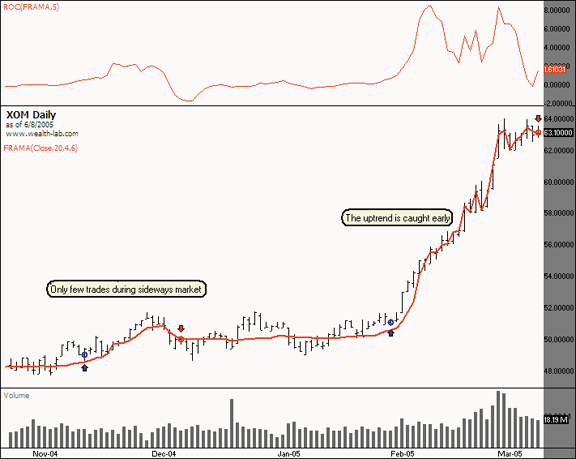FIGURE 4: WEALTH-LAB, FRACTAL ADAPTIVE MOVING AVERAGES. The ExxonMobil price series together with its 20-day FRAMA is plotted in the lower pane. The upper pane shows the rate of change (ROC) of five days of the FRAMA indicator. During sideways phases, the FRAMA indicator shows only little movement. Consequently, the ROC shows small values and only few trades occur. At the end of January 2005, a strong uptrend starts, which is detected by the FRAMA. The system is able to enter early and catches most of this upmove.
```WealthScript Code:
{\$I 'FRAMA'}
var Bar, ROCPane: integer;
var F:integer = FRAMASeries(#Close, 20, 4.6);
var R:integer = ROCSeries(F, 5);
PlotSeriesLabel(F, 0, #red, #thick, 'FRAMA(Close,20,4.6)');
ROCPane:=CreatePane(75,true,true);
PlotSeriesLabel(R, ROCPane, #red, #thin,'ROC(FRAMA,5)');
for Bar := 20 to BarCount - 1 do
begin
if not LastPositionActive then
begin
if @R[Bar] > 0.5 then BuyAtMarket(Bar+1, 'entry signal');
end
else
begin
if @R[Bar] < 0 then SellAtMarket(Bar+1, LastPosition, 'exit signal');
end;
end;```
-- José Cruset, Wealth-Lab, Inc.
www.wealth-lab.com
GO BACK

For this issue's article by John Ehlers, "Fractal Adaptive Moving Averages," we've provided the eSignal formula file named "Frama.efs." The code is also displayed here.

The study has one parameter for the length, or periods, for the study that may be adjusted through the "Edit Studies" option of the Advanced Chart. The number entered will be forced to be the next highest even number if an odd number is entered. A sample eSignal chart is shown in Figure 5.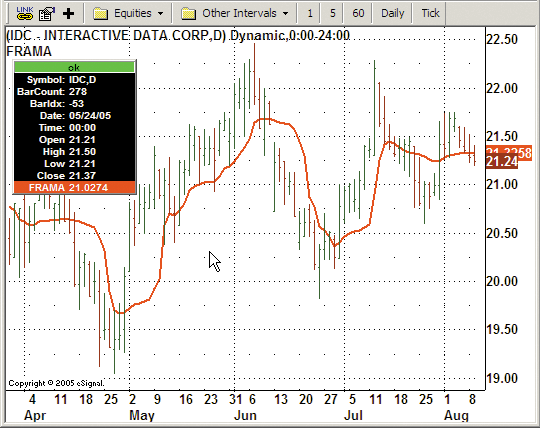FIGURE 5: eSIGNAL, FRACTAL ADAPTIVE MOVING AVERAGE. This eSignal chart demonstrates the fractal adaptive moving average.
```/***************************************
Provided By : eSignal (c) Copyright 2005
Description:  Fractal Adaptive Moving Average - by John Ehlers
Version 1.0  8/9/2005
Notes:
October 2005 Issue - "FRAMA - Fractal Adaptive Moving Average"
* Study requires version 7.9 or higher.
* Length will be forced to be an even number. Odd numbers will be bumped up to the
next even number.
Formula Parameters:     Defaults:
Length                              16
***************************************/
function preMain() {
setPriceStudy(true);
setStudyTitle("FRAMA ");
setShowTitleParameters(false);
setCursorLabelName("FRAMA", 0);
setDefaultBarFgColor(Color.red, 0);
setDefaultBarThickness(2, 0);

var fp1 = new FunctionParameter("nLength", FunctionParameter.NUMBER);
fp1.setName("Length");
fp1.setDefault(16);
fp1.setLowerLimit(1);
}
var bVersion = null;
var Filt = null;
var Filt_1 = null;   //previous bar's Filt
function main(nLength) {
if (bVersion == null) bVersion = verify();
if (bVersion == false) return;
var nState = getBarState();

if (nState == BARSTATE_NEWBAR) {
Filt_1 = Filt;
}

var N = Math.round(nLength/2) * 2; // forces N to be even number
var Price = hl2();
var count = 0;
var N1 = 0;
var N2 = 0;
var N3 = (highest(N, high()) - lowest(N, low())) / N;
var HH = high(0);
var LL = low(0);
var Dimen = 0;
var alpha = 0;
Filt = 0;

if (Filt_1 == null) Filt_1 = 0;

for( count = 0; count <= (N/2 -1); count++) {
if (high(-count) > HH) HH = high(-count);
if (low(-count) < LL) LL = low(-count);
}
N1 = (HH - LL) / (N / 2);
HH = high(-(N/2));
LL = low(-(N/2));

for (count = (N/2); count <= (N-1); count++) {
if (high(-count) > HH) HH = high(-count);
if (low(-count) < LL) LL = low(-count);
}
N2 = (HH - LL) / (N / 2);

if (N1 > 0 && N2 > 0 && N3 > 0) {
Dimen = (Math.log(N1 + N2) - Math.log(N3)) / Math.log(2);
}

alpha = Math.exp(-4.6*(Dimen - 1));

if (alpha < 0.01) alpha = 0.01;
if (alpha > 1) alpha = 1;

Filt = (alpha*Price) + (1 - alpha)*Filt_1;

if (getCurrentBarCount() < N) Filt = Price;
return Filt;
}
/***** Support Functions *****/
function verify() {
var b = false;
if (getBuildNumber() < 700) {
drawTextAbsolute(5, 35, "This study requires version 7.9 or later.", Color.white,
Color.blue, Text.RELATIVETOBOTTOM|Text.RELATIVETOLEFT|Text.BOLD|Text.LEFT,
null, 13, "error");
return b;
} else {
b = true;
}
return b;
}```

To discuss this study or download a complete copy of the formula, please visit the Efs Library Discussion Board forum under the Bulletin Boards link at www.esignalcentral.com. This eSignal formula code is also available for copying and pasting from the STOCKS & COMMODITIES website at Traders.com.

--Jason Keck
eSignal, a division of Interactive Data Corp.
800 815-8256, www.esignal.com
GO BACK

The fractal adaptive moving average introduced by John Ehlers in this issue can be easily implemented in NeuroShell Trader by combining a few of NeuroShell Trader's 800+ indicators and one custom indicator, which in itself is a very useful generic adaptive moving average.

To implement the fractal adaptive moving average, select "New Indicator ..." from the Insert menu and use the Indicator Wizard to create the following indicators:

```N3:  Divide( Subtract( PriceRange( High, Low, n ), n )
N1:  Divide( Subtract( PriceRange( High, Low, n/2 ), n/2 )
N2:  Lag( N1, n/2 )
Dimen :  Divide( Subtract( Log( Add2( N1, N2 ), Log(N3), Log(2) )
Alpha:  Min2( Max2( Exp ( Multiply ( -4.6, Subtract ( Dimen, 1 ) ) ), .01), 1 )
Filt: AdaptiveExpAvg* ( Avg2( High, Low ), Alpha )```
Users of NeuroShell Trader can go to the STOCKS & COMMODITIES section of the NeuroShell Trader free technical support website to download custom indicators and a sample chart (Figure 6).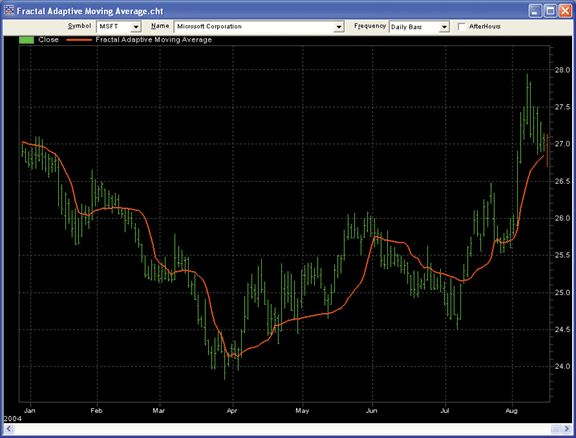FIGURE 6: NEUROSHELL TRADER, FRAMA. Here's a sample NeuroShell Trader chart demonstrating the fractal adaptive moving average.

--Marge Sherald, Ward Systems Group, Inc.
301 662-7950, sales@wardsystems.com
www.neuroshell.com
GO BACK

In "Fractal Adaptive Moving Averages," John Ehlers presents a new method of adaptive smoothing based on the assumption that market prices are fractal. Coding the fractal adaptive moving average (FRAMA) is relatively straightforward in AmiBroker Formula Language (AFL). Thanks to its powerful array-processing functions, FRAMA can be implemented in AmiBroker without any loops, making it extremely fast.

Ready-to-use code is presented in Listing 1. For comparison purposes, the code also plots a standard exponential moving average of the same length (Figure 7).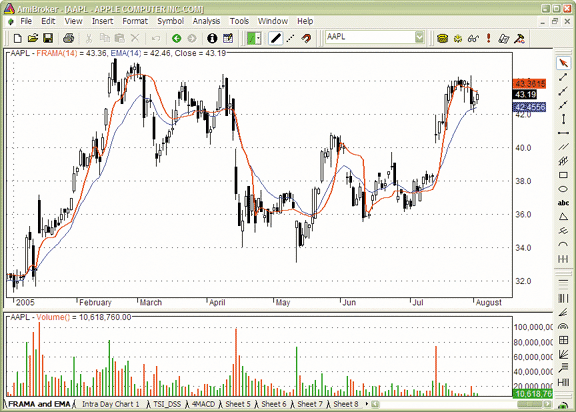FIGURE 7: AMIBROKER, FRACTAL ADAPTIVE MOVING AVERAGE. This AmiBroker screenshot shows a price chart of AAPL with a 14-day FRAMA (red line) and exponential moving average (blue line) of the same length. FRAMA follows significant changes in price more rapidly, while maintaining smoothness in congestion zones.

LISTING 1
// FRAMA - Fractal Adaptive Moving Average
Price = (H+L)/2;
N = Param( "N", 16, 2, 40, 2 ); // must be even

N3 = ( HHV( High, N ) - LLV( Low, N ) ) / N;

HH = HHV( High, N / 2 ) ;
LL = LLV( Low, N / 2 );

N1 = ( HH - LL ) / ( N / 2 );

HH = HHV( Ref( High, - N/2 ), N/2 );
LL = LLV( Ref( Low, - N/2 ), N/ 2 );

N2 = ( HH - LL ) / ( N / 2 );

Dimen = IIf( N1 > 0 AND N2 > 0 AND N3 > 0, ( log( N1+N2) - log( N3 ) )/log( 2 ), Null );

alpha = exp( -4.6 * (Dimen -1 ) );
alpha = Min( Max( alpha, 0.01 ), 1 ); // bound to 0.01...1 range

Frama = AMA( Price, alpha );

Plot( Frama, "FRAMA("+N+")", colorRed, styleThick );
Plot( EMA( C, N ) , "EMA("+N+")", colorBlue );
Plot( C, "Close", colorBlack, styleCandle );

--Tomasz Janeczko, AmiBroker.com
www.amibroker.com
GO BACK

The fractal adaptive moving average (FRAMA) computation presented in the article "Fractal Adaptive Moving Averages" by John Ehlers can be implemented as a NeoTicker indicator. Listing 1 shows the code for the fractal adaptive moving average indicator, with two parameters. The first parameter is price, which is a formula parameter that uses the average price calculation as the default. The second parameter is N, which is an integer parameter with 16 as the default.

The NeoTicker fractal adaptive moving average indicator plots a line that connects the calculation result of a fractal average for each bar. This indicator, like any other indicator, can be used in a trading system, as shown in the sample chart in Figure 8, where a crossover system is constructed using FRAMA.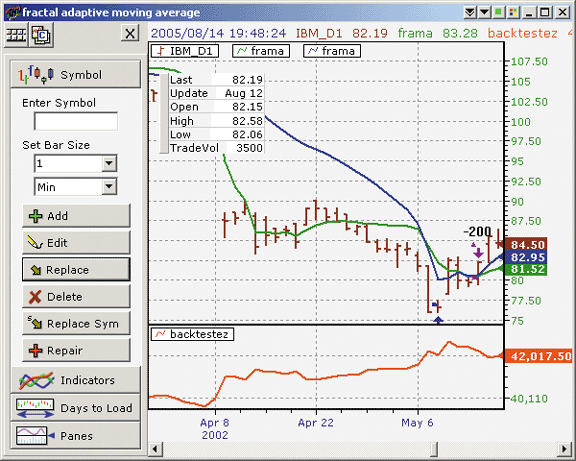FIGURE 8: NEOTICKER, FRACTAL ADAPTIVE MOVING AVERAGE. Here's a sample NeoTicker chart showing a crossover system constructed using the FRAMA indicator.

A downloadable version of this indicator and sample chart will be available at the NeoTicker Yahoo! User Group.

```LISTING 1
function frama()
Const Filt = 0
N    = 1
dim myhhv, myllv, myprice
myhhv = itself.makeindicator ("hhv1", "hhv", Array("1.h"), _
Array(params("N").str))
myllv = itself.makeindicator ("llv1", "llv", Array("1.l"), _
Array(params("N").str))
myprice = itself.makeindicator ("price1", "fml", Array("1"), _
Array(params("Price").str))
if heap.size = 0 then
heap.allocate(2)
heap.value(Filt) = 0
heap.value(N) = params("N").int
end if
if data1.barsnum(0) < (heap.value(N)+1) then
itself.success = false
heap.value(Filt) = myprice.value(0)
exit function
end if
if (heap.value(N) mod 2) <> 0 then
ntlib.debug("N must be even number, calculation abort")
itself.success = false
exit function
end if
N3 = (myhhv.value(0)-myllv.value(0))/heap.value(N)
HH = data1.high(0)
LL = data1.low(0)
for i=0 to heap.value(N)/2-1
if data1.high(i) > HH then
HH = data1.high(i)
end if
if data1.low(i) < LL then
LL = data1.low(i)
end if
next
N1 = (HH-LL)/(heap.value(N)/2)
HH = data1.high(heap.value(N)/2)
LL = data1.low(heap.value(N)/2)
for i=heap.value(N)/2 to heap.value(N)-1
if data1.high(i) > HH then
HH = data1.high(i)
end if
if data1.low(i) < LL then
LL = data1.low(i)
end if
next
N2 = (HH-LL)/(heap.value(N)/2)
if N1>0 and N2>0 and N3>0 then
Dimen = (ntlib.ln(N1+N2) - ntlib.ln(N3))/ntlib.ln(2)
alpha = ntlib.exp(-4.6*(Dimen-1))
end if
if alpha<0.01 then alpha = 0.01
if alpha>1 then alpha = 1
frama = alpha*myprice.value(0)+(1-alpha)*heap.value(Filt)
heap.value(Filt) = frama
end function```

--Kenneth Yuen, TickQuest Inc.
www.tickquest.com

GO BACK

In his article "Fractal Adaptive Moving Averages," John Ehlers describes an exponential moving average based on recent volatility, using fractal dimensions of recent prices to establish an alpha.

```This code can be entered into TradingSolutions as follows:
Name: FRAMA Boxes
Inputs: High, Low, Period, Maximum Period
Div (Sub (HighestVL (High, Period, Maximum Period), LowestVL (Low, Period,
Maximum Period)), Period)
Name: FRAMA Dimension
Inputs: High, Low, Period
Div (Sub (Log10 (Add (FRAMABoxes (High, Low, Div (Period, 2),Period), LagVL
(FRAMABoxes (High, Low, Div (Period, 2), Period),Div (Period, 2), Period))),
Log10 (FRAMABoxes (High, Low, Ident (Period), Period))), Log10 (2))
Name: FRAMA Alpha
Inputs: High, Low, Period
Max (Min (Exp (Mult (-4.6, Sub (FRAMADimension (High, Low, Period),1))),1),0.01)
Name: FRAMA
Inputs: High, Low, Period
EMA%% (Avg (High, Low), FRAMAAlpha (High, Low, Period))```

This function is also available as a downloadable file from the TradingSolutions website (www.tradingsolutions.com) in the Solution Library section. As with many indicators, this function could make a good input to neural network predictions.

--Gary Geniesse, NeuroDimension, Inc.
800 634-3327, 352 377-5144
GO BACK

FINANCIAL DATA CALCULATOR: Fractal Adaptive Moving Average

The article "Fractal Adaptive Moving Averages" by John Ehlers shows how to use a fractal dimension approximation to make an exponential moving average adaptive. In Financial Data Calculator (FDC), this is most easily done using three macros:

```1. boxcount:
@ boxcount computes the boxcount each day for
@John Ehlers' Fractal Adaptive Moving Average
@syntax is 'n boxcount dataset' (n even)
N: #L
DS: #R
HH: N MOVMAX HIGH DS
LL: N MOVMIN LOW DS
(HH-LL)/N
Example: 10 BOXCOUNT IBM
2. fracdim:
@fracdim computes the fractal dimension each day
@for John Ehlers' Fractal Adaptive Moving Average
@syntax is 'n fracdim dataset' (n even)
N: #L
DS: #R
N3: N BOXCOUNT DS
N2: (N/2) BOXCOUNT DS
N1: (N/2) BOXCOUNT DS  BACK N/2
((LOG (N1 + N2)) -LOG N3)/LOG 2
Example: 10 FRACDIM IBM
3. frama:
@John Ehlers' Fractal Adaptive Moving Average
@syntax is 'n frama dataset'
N: #L
DS: #R
H: HIGH DS
L: LOW DS
ALPHA: EXP (-4.6 * (-1 + N FRACDIM DS ))
ALPHA EXPAVE (H + L)/2
Example: 10 FRAMA IBM```

--Bill Rafter
Mathematical Investment Decisions Inc.
856 857-9088, mathinvestdecisions.com

GO BACK